FunWithElectronics.com- Collection of Information for those with Electronics as a Hobby
Up one level (GNU Radio and USRP)

## TV Receiver with audio using USRP

Want to use your USRP to watch analog television? Here is a modified usrp_tv_rcv.py script which takes advantage of several channels in the USRP to also receive the audio. For the moment it's a CPU hog, but as long as you are not doing anything else on your (not too slow) computer, it will run smoothly.

usrp_tv_with_sound.py

Below is also shown how little code is needed in order to get some frames from the analog television channel.

```#!/usr/bin/env python

import sys

frekvens = float(sys.argv)*1000000-5.5e6 #161.25e6 # animal planet

fg = gr.top_block()
u = usrp.source_c()
u.set_decim_rate(32)
subdev_spec = (1,0)

u.set_mux(usrp.determine_rx_mux_value(u,subdev_spec))
subdev = usrp.selected_subdev(u,subdev_spec)
subdev.set_gain(50)
u.tune(0,subdev,frekvens)

hode = gr.head(gr.sizeof_gr_complex,2*625*80)  # 625 linjer
filut = gr.file_sink(gr.sizeof_gr_complex,"/lager/ruben/tvut.raw")

fg.connect(u, hode, filut)

fg.run()
```

### MATLAB-code for plotting the images

```clear all;
fid = fopen('\\192.168.1.50\lager\ruben\tvut.raw','r');
fclose(fid);
x1 = x(1:2:length(x));
x2 = x(2:2:length(x));
x = complex(x1,x2);
x = x(12000:length(x));  % Skipper begynnelse
x = x(1:floor(length(x)/128)*128);  % hele antall linjer
xabs = abs(x);
xabsinvertert = max(xabs)-xabs;
plot(xabsinvertert)     % decim 32 gir rate 2MHz, en frame: 80000 samples
% 625 linjer gir: 128 samples på hver linje

xabsinvertert = xabsinvertert./(max(xabsinvertert));
svarthvit = reshape(xabsinvertert,128,length(x)/128)'; % linjeskift
rgbversjon=cat(3,svarthvit,svarthvit,svarthvit);
image(rgbversjon)
```

### Results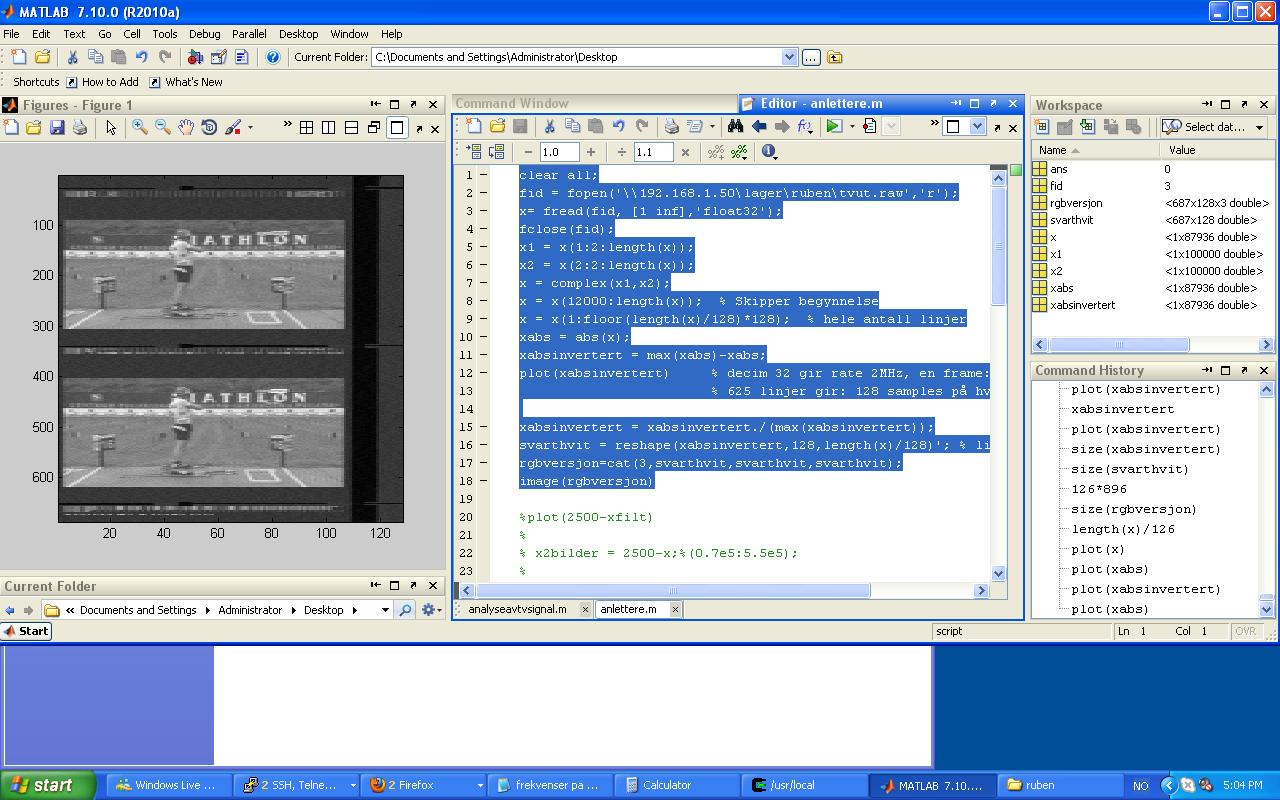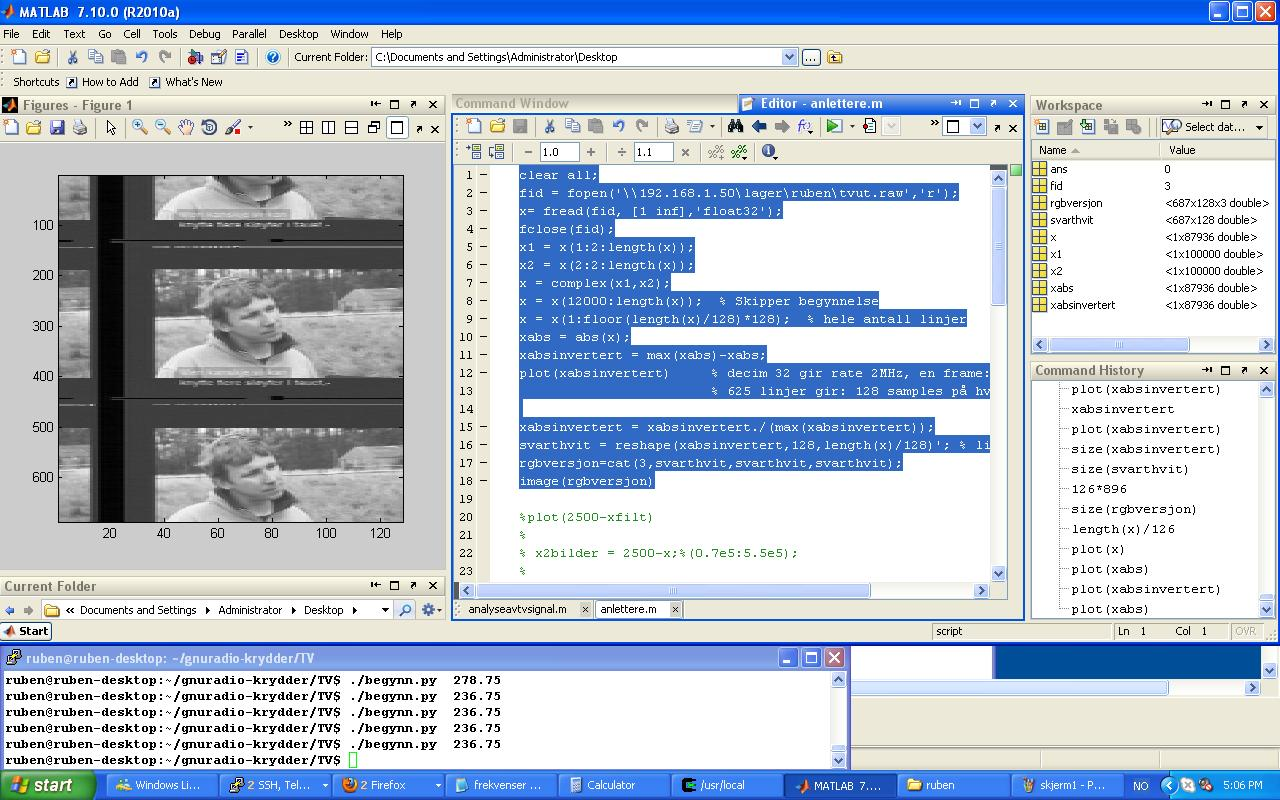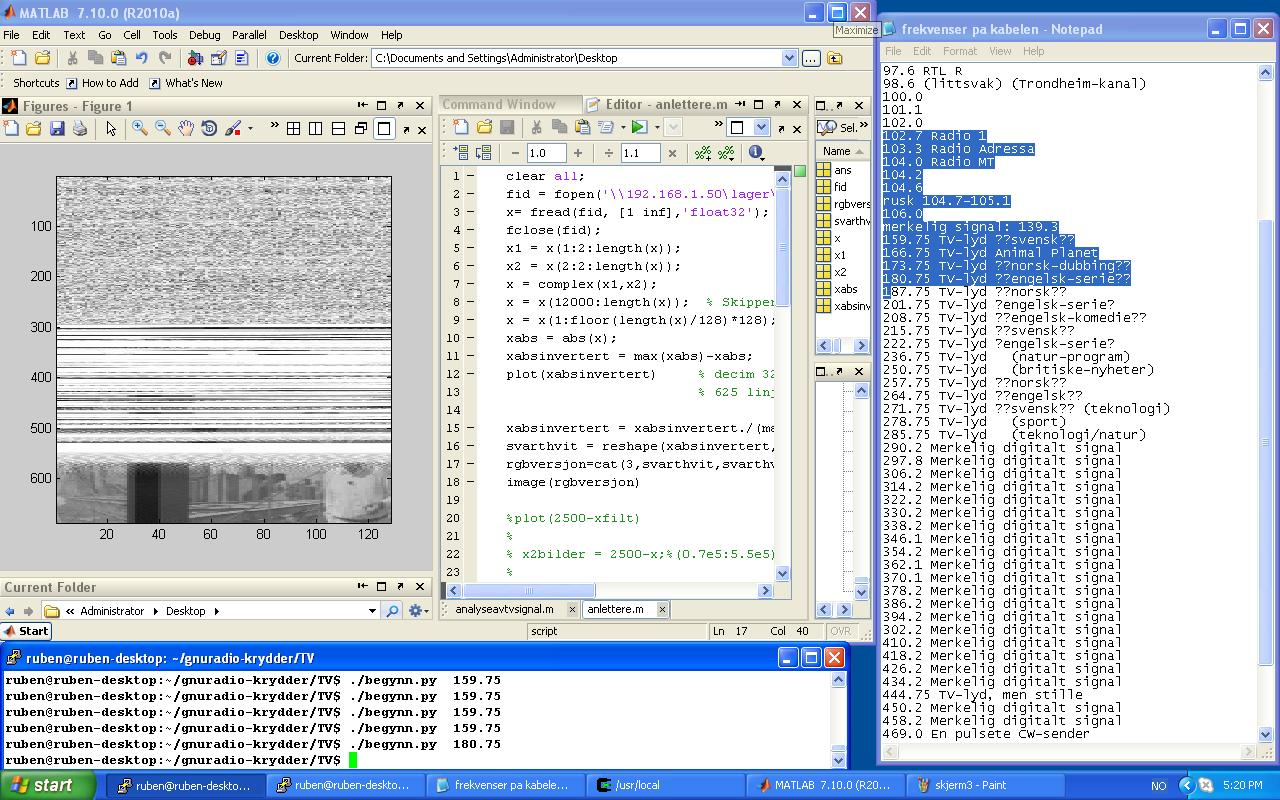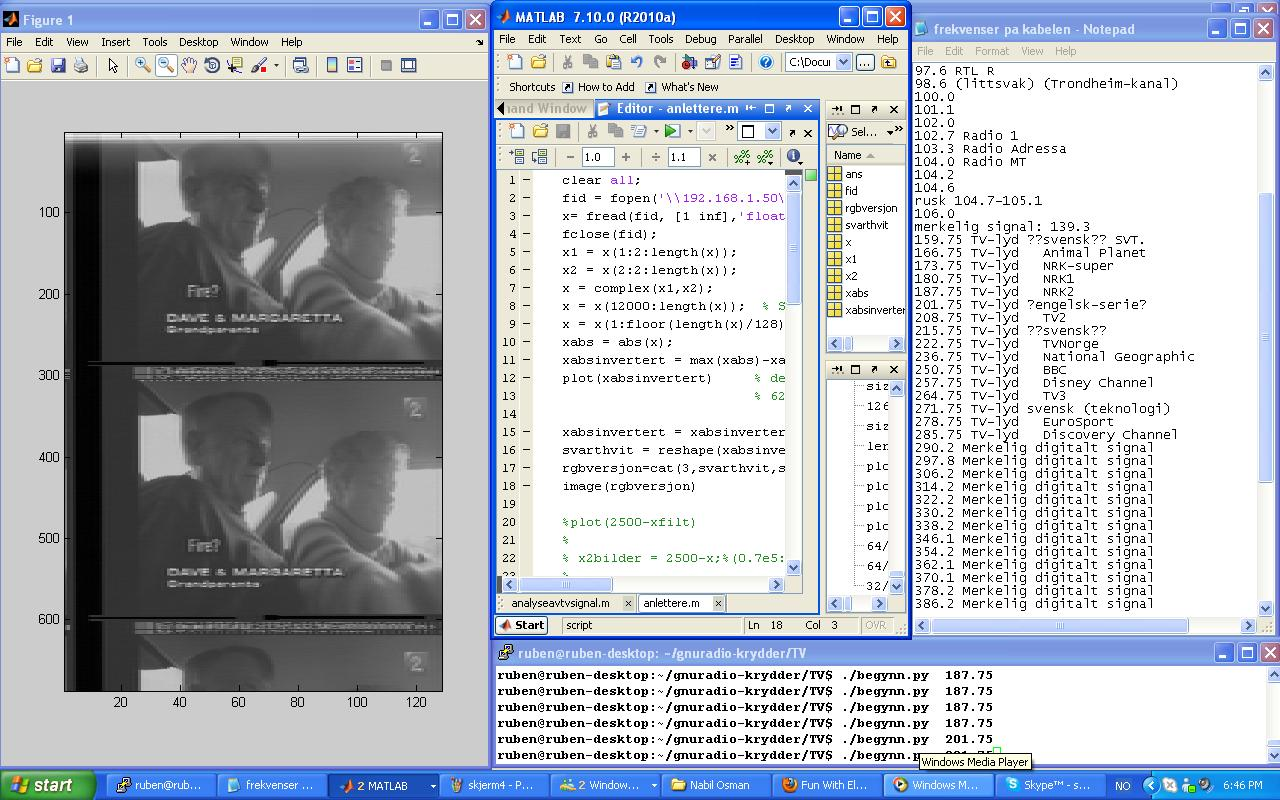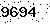Fill in number